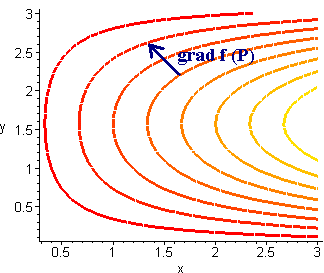# 1.10: The Gradient

$$\newcommand{\vecs}{\overset { \rightharpoonup} {\mathbf{#1}} }$$ $$\newcommand{\vecd}{\overset{-\!-\!\rightharpoonup}{\vphantom{a}\smash {#1}}}$$$$\newcommand{\id}{\mathrm{id}}$$ $$\newcommand{\Span}{\mathrm{span}}$$ $$\newcommand{\kernel}{\mathrm{null}\,}$$ $$\newcommand{\range}{\mathrm{range}\,}$$ $$\newcommand{\RealPart}{\mathrm{Re}}$$ $$\newcommand{\ImaginaryPart}{\mathrm{Im}}$$ $$\newcommand{\Argument}{\mathrm{Arg}}$$ $$\newcommand{\norm}{\| #1 \|}$$ $$\newcommand{\inner}{\langle #1, #2 \rangle}$$ $$\newcommand{\Span}{\mathrm{span}}$$ $$\newcommand{\id}{\mathrm{id}}$$ $$\newcommand{\Span}{\mathrm{span}}$$ $$\newcommand{\kernel}{\mathrm{null}\,}$$ $$\newcommand{\range}{\mathrm{range}\,}$$ $$\newcommand{\RealPart}{\mathrm{Re}}$$ $$\newcommand{\ImaginaryPart}{\mathrm{Im}}$$ $$\newcommand{\Argument}{\mathrm{Arg}}$$ $$\newcommand{\norm}{\| #1 \|}$$ $$\newcommand{\inner}{\langle #1, #2 \rangle}$$ $$\newcommand{\Span}{\mathrm{span}}$$$$\newcommand{\AA}{\unicode[.8,0]{x212B}}$$

## Directional Derivatives

Suppose you are given a topographical map and want to see how steep it is from a point that is neither due West or due North. Recall that the slopes due north and due west are the two partial derivatives. The slopes in other directions will be called the directional derivatives. Formally, we define

Definition: Directional Derivatives

Let $$f(x,y)$$ be a differentiable function and let u be a unit vector then the directional derivative of $$f$$ in the direction of u is

$D_u f(x,y) =\lim_{t \rightarrow 0} \dfrac{f(x+tu_1,y+tu_2) - f(x,y) }{t}. \nonumber$

Note that if u is $$\hat{\textbf{i}}$$ then the directional derivative is just $$f_x$$ and if u is $$\hat{\textbf{i}}$$ the it is $$f_y$$. Just as there is a difficult and an easy way to compute partial derivatives, there is a difficult way and an easy way to compute directional derivatives.

Let $$f(x,y)$$ be a differentiable function, and u be a unit vector with direction $$\hat{\textbf{q}}$$, then

$D_u f(x,y) = \left \langle f_x,f_y \right \rangle \cdot \left \langle \cos \theta, \sin \theta \right \rangle .\nonumber$

Example $$\PageIndex{1}$$

Let

$f(x,y) = 2x + 3y^2 - xy \nonumber$

and

$\textbf{v} = \left \langle 3,2 \right \rangle .\nonumber$

Find

$D_v f(x,y) .\nonumber$

Solution

We have

$f_x = 2 - y \;\;\; \text{and} \;\;\; f_y = 6y - x \nonumber$

and

$|| \textbf{v} || = \sqrt{9+4} = \sqrt{13} . \nonumber$

Hence

\begin{align*} D_v f(x,y) &= \left \langle 2 - y, 6y - x \right \rangle \cdot \left \langle \frac{3}{\sqrt{13}}, \frac{2}{\sqrt{13}} \right \rangle \\ &= \dfrac{2}{\sqrt{13}} (2-y) + \dfrac{3}{\sqrt{13}} (6y-x). \end{align*}

Exercise $$\PageIndex{1}$$

Let

$f(x,y)= e^{xy^2} \;\;\; and \;\;\; v=\langle2,-5\rangle . \nonumber$

Find $$D_v \; f(x,y)$$

We define

$\nabla f = \langle f_x,f_y\rangle . \nonumber$

Notice that

$D_u f(x,y) = (\nabla f) \cdot u .\nonumber$

The gradient has a special place among directional derivatives. The theorem below states this relationship.

Theorem

1. If $$\nabla f(x,y) = 0$$ then for all u, $$D_u f(x,y) = 0$$.
2. The direction of $$\nabla f(x,y)$$ is the direction with maximal directional derivative.
3. The direction of $$\nabla f(x,y)$$ is the direction with the minimal directional derivative.

Proof

1. If

$\nabla f(x,y) = 0\nonumber$

then

$D_u f(x,y) = \nabla f \cdot \textbf{u} = 0 \cdot \textbf{u} = 0. \nonumber$

2.

$D_u f(x,y) = \nabla f \cdot \textbf{u} = || \nabla f || \cos q. \nonumber$

This is a maximum when $$q = 0$$ and a minimum when $$q = p$$. If $$q = 0$$ then $$\nabla f$$ and u point in the same direction. If $$q = p$$ then u and $$\nabla f$$ point in opposite directions. This proves 2 and 3.

Example $$\PageIndex{2}$$

Suppose that a hill has altitude

$w(x,y) = x^2 - y .\nonumber$

Find the direction that is the steepest uphill and the steepest downhill at the point $$(2,3)$$.

Solution

We find

$\nabla w = \langle 2x, -y\rangle = \langle 4, -3\rangle .\nonumber$

Hence the steepest uphill is in the direction

$\langle 4,-3\rangle \nonumber$

while the steepest downhill is in the direction

$-\langle 4,-3\rangle = \langle -4,3\rangle .\nonumber$

## The Gradient and Level Curves

If $$f$$ is differentiable at $$(a,b)$$ and $$\nabla f$$ is nonzero at $$(a,b)$$ then $$\nabla$$ is perpendicular to the level curve through $$(a,b)$$.## Contributors and Attributions

This page titled 1.10: The Gradient is shared under a not declared license and was authored, remixed, and/or curated by Larry Green.